Скачать презентацию Rates Warm Up Problem of the Day Lesson

89d5ef6d0d30e59acaf064358b8beba5.ppt

• Количество слайдов: 23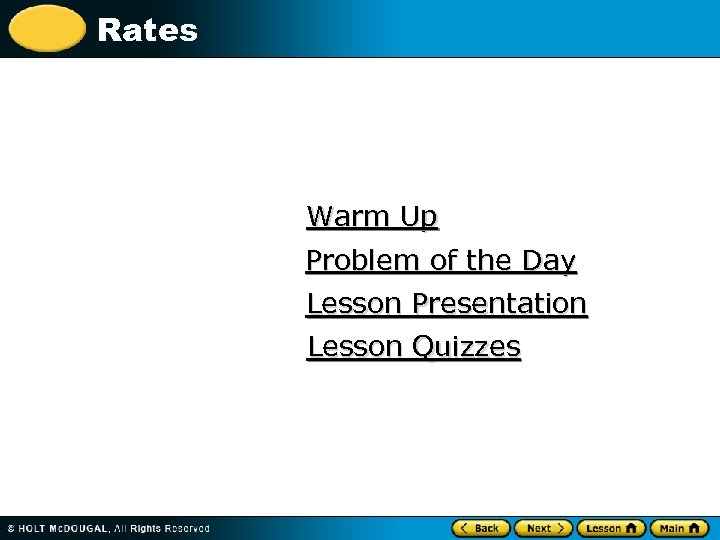Rates Warm Up Problem of the Day Lesson Presentation Lesson Quizzes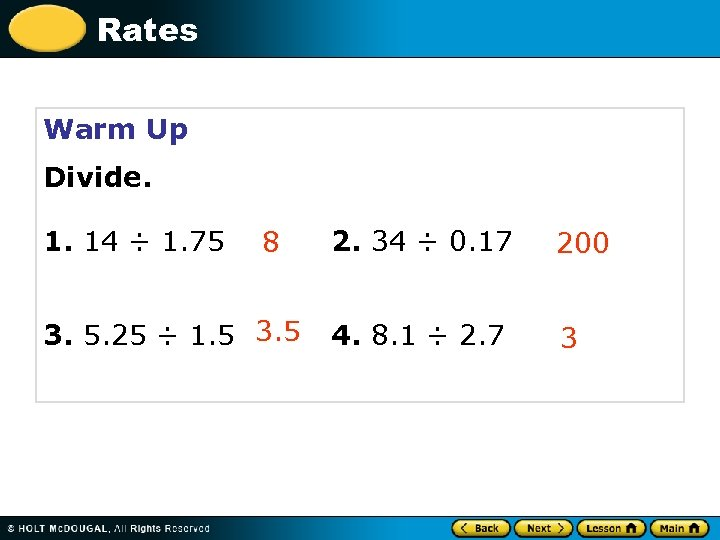Rates Warm Up Divide. 1. 14 ÷ 1. 75 8 3. 5. 25 ÷ 1. 5 3. 5 2. 34 ÷ 0. 17 200 4. 8. 1 ÷ 2. 7 3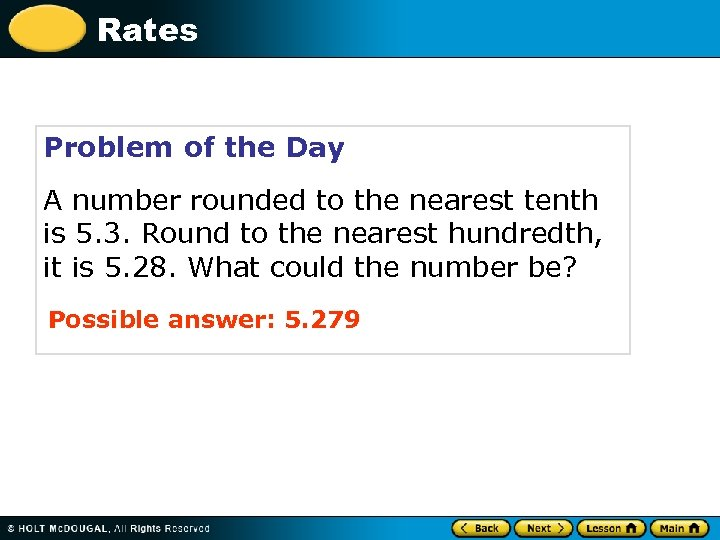Rates Problem of the Day A number rounded to the nearest tenth is 5. 3. Round to the nearest hundredth, it is 5. 28. What could the number be? Possible answer: 5. 279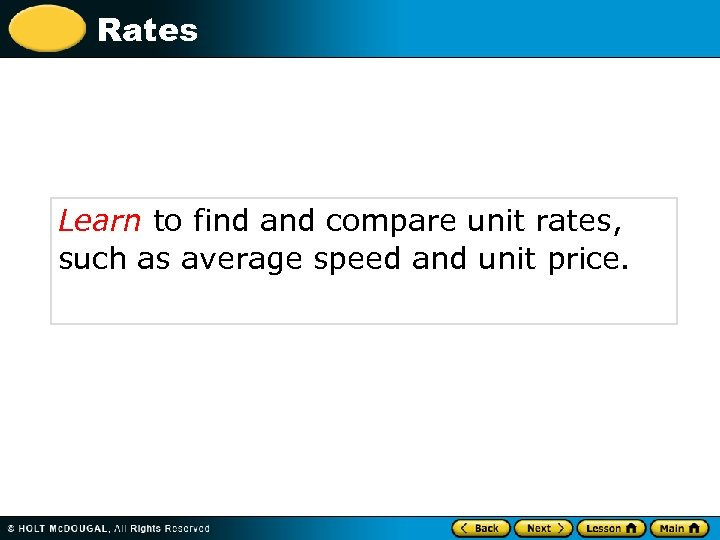Rates Learn to find and compare unit rates, such as average speed and unit price.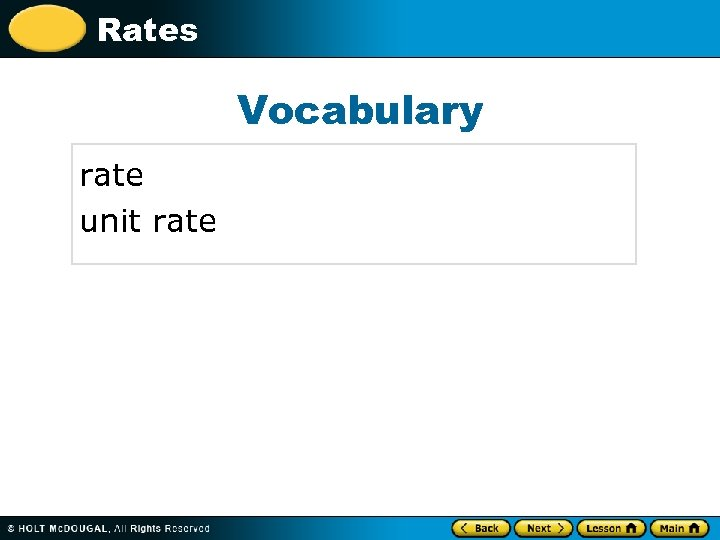Rates Vocabulary rate unit rate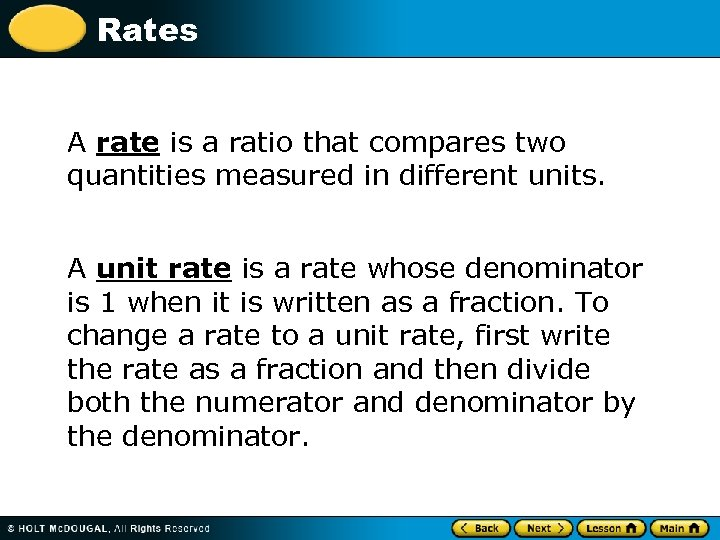Rates A rate is a ratio that compares two quantities measured in different units. A unit rate is a rate whose denominator is 1 when it is written as a fraction. To change a rate to a unit rate, first write the rate as a fraction and then divide both the numerator and denominator by the denominator.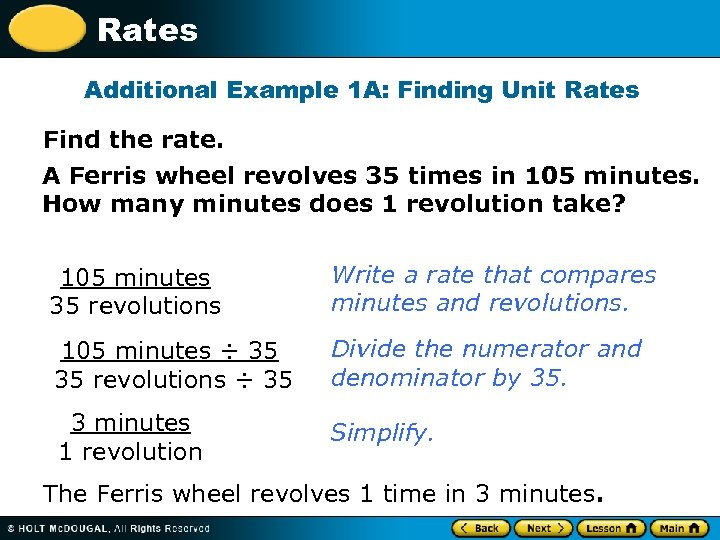Rates Additional Example 1 A: Finding Unit Rates Find the rate. A Ferris wheel revolves 35 times in 105 minutes. How many minutes does 1 revolution take? 105 minutes 35 revolutions Write a rate that compares minutes and revolutions. 105 minutes ÷ 35 35 revolutions ÷ 35 Divide the numerator and denominator by 35. 3 minutes 1 revolution Simplify. The Ferris wheel revolves 1 time in 3 minutes.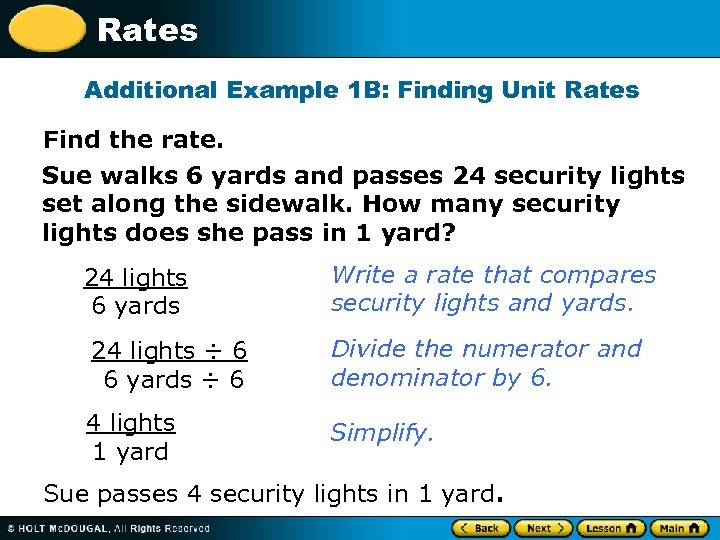Rates Additional Example 1 B: Finding Unit Rates Find the rate. Sue walks 6 yards and passes 24 security lights set along the sidewalk. How many security lights does she pass in 1 yard? 24 lights 6 yards Write a rate that compares security lights and yards. 24 lights ÷ 6 6 yards ÷ 6 Divide the numerator and denominator by 6. 4 lights 1 yard Simplify. Sue passes 4 security lights in 1 yard.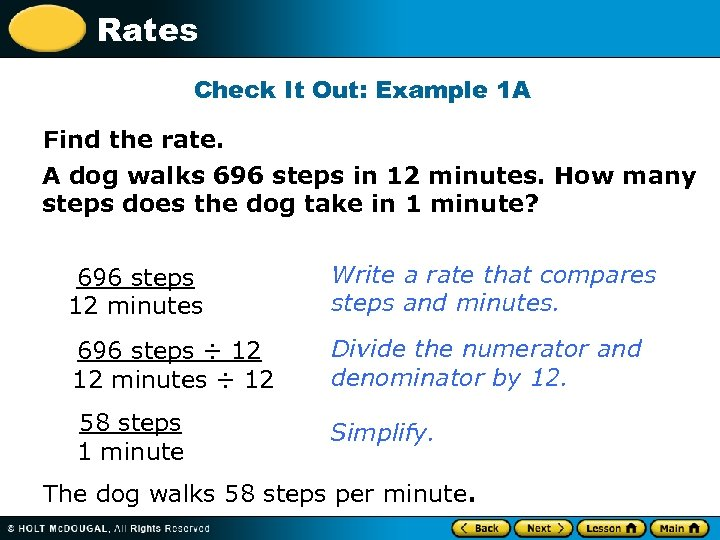Rates Check It Out: Example 1 A Find the rate. A dog walks 696 steps in 12 minutes. How many steps does the dog take in 1 minute? 696 steps 12 minutes Write a rate that compares steps and minutes. 696 steps ÷ 12 12 minutes ÷ 12 Divide the numerator and denominator by 12. 58 steps 1 minute Simplify. The dog walks 58 steps per minute.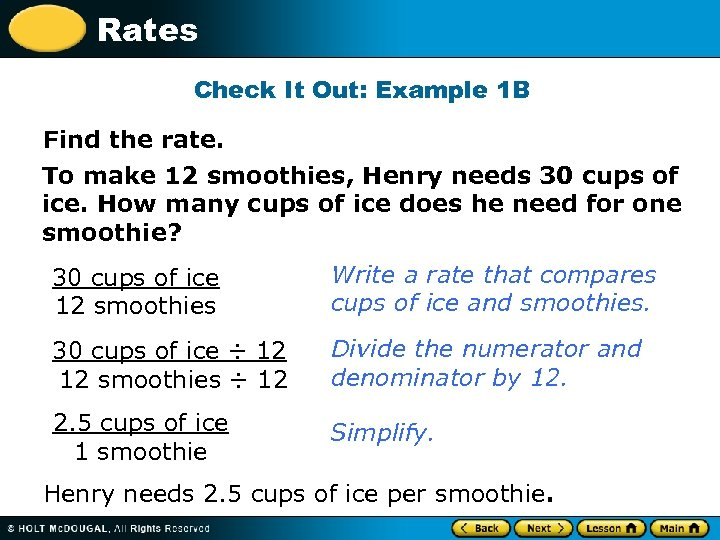Rates Check It Out: Example 1 B Find the rate. To make 12 smoothies, Henry needs 30 cups of ice. How many cups of ice does he need for one smoothie? 30 cups of ice 12 smoothies Write a rate that compares cups of ice and smoothies. 30 cups of ice ÷ 12 12 smoothies ÷ 12 Divide the numerator and denominator by 12. 2. 5 cups of ice 1 smoothie Simplify. Henry needs 2. 5 cups of ice per smoothie.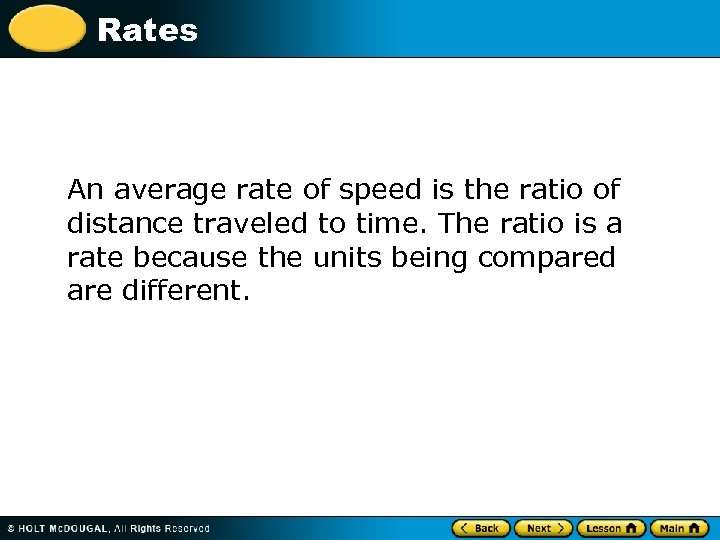Rates An average rate of speed is the ratio of distance traveled to time. The ratio is a rate because the units being compared are different.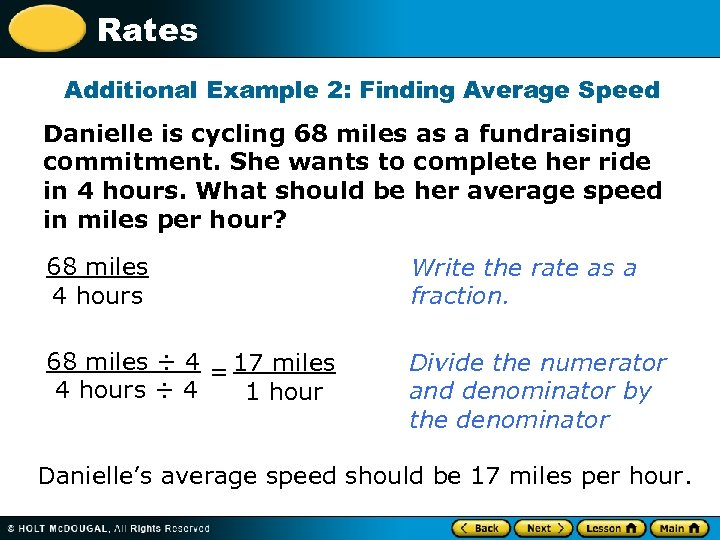Rates Additional Example 2: Finding Average Speed Danielle is cycling 68 miles as a fundraising commitment. She wants to complete her ride in 4 hours. What should be her average speed in miles per hour? 68 miles 4 hours Write the rate as a fraction. 68 miles ÷ 4 = 17 miles 4 hours ÷ 4 1 hour Divide the numerator and denominator by the denominator Danielle’s average speed should be 17 miles per hour.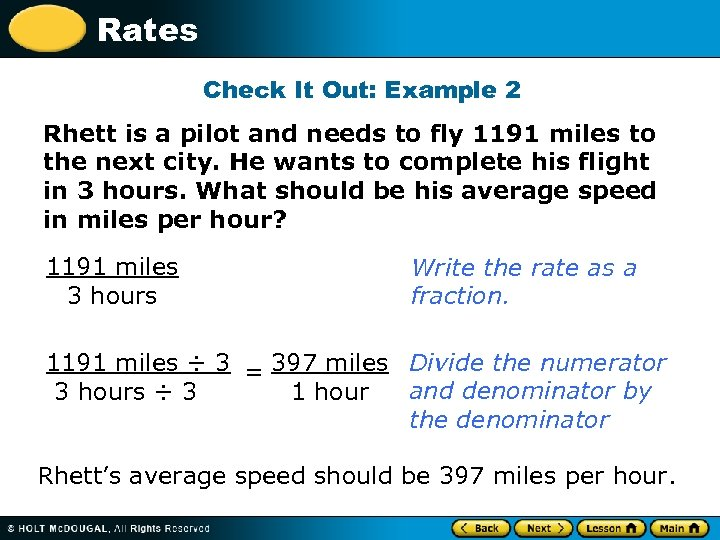Rates Check It Out: Example 2 Rhett is a pilot and needs to fly 1191 miles to the next city. He wants to complete his flight in 3 hours. What should be his average speed in miles per hour? 1191 miles 3 hours Write the rate as a fraction. 1191 miles ÷ 3 = 397 miles Divide the numerator and denominator by 3 hours ÷ 3 1 hour the denominator Rhett’s average speed should be 397 miles per hour.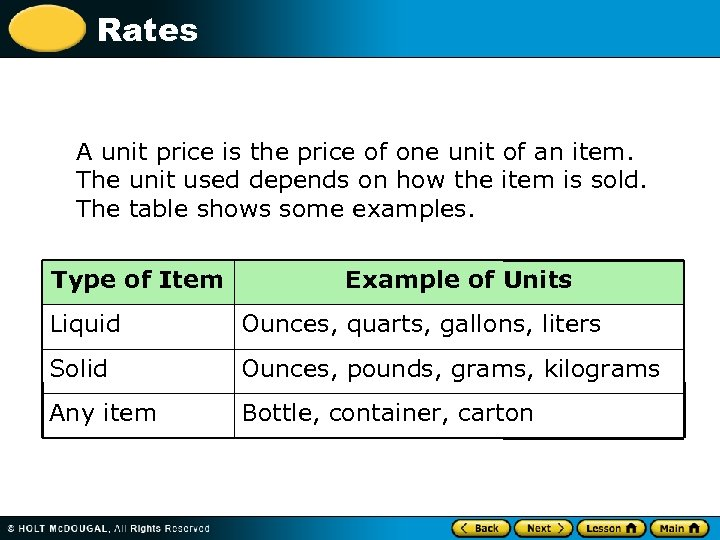Rates A unit price is the price of one unit of an item. The unit used depends on how the item is sold. The table shows some examples. Type of Item Example of Units Liquid Ounces, quarts, gallons, liters Solid Ounces, pounds, grams, kilograms Any item Bottle, container, carton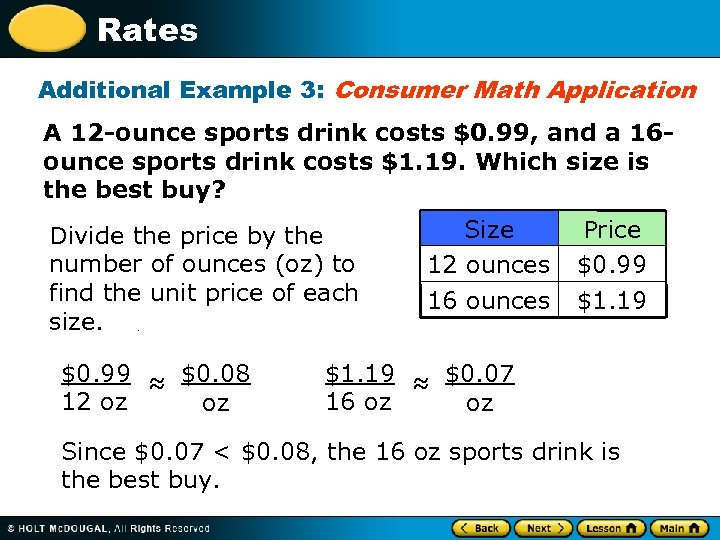Rates Additional Example 3: Consumer Math Application A 12 -ounce sports drink costs \$0. 99, and a 16 ounce sports drink costs \$1. 19. Which size is the best buy? Divide the price by the number of ounces (oz) to find the unit price of each size. \$0. 99 ≈ \$0. 08 12 oz oz Size 12 ounces 16 ounces Price \$0. 99 \$1. 19 ≈ \$0. 07 16 oz oz Since \$0. 07 < \$0. 08, the 16 oz sports drink is the best buy.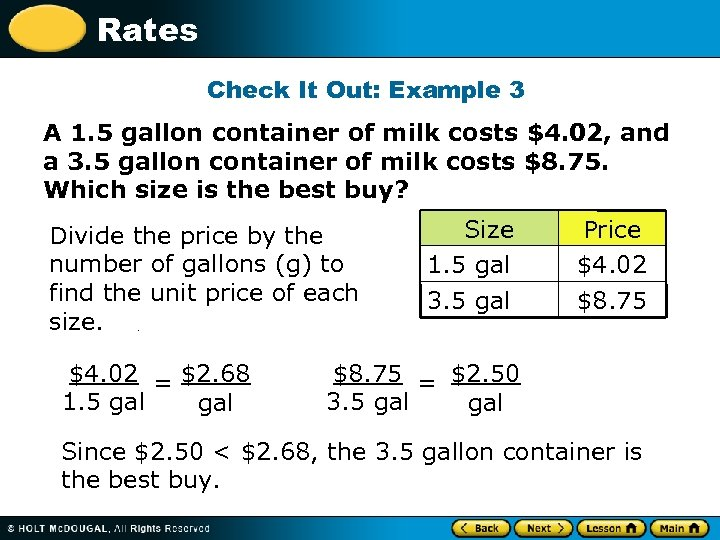Rates Check It Out: Example 3 A 1. 5 gallon container of milk costs \$4. 02, and a 3. 5 gallon container of milk costs \$8. 75. Which size is the best buy? Divide the price by the number of gallons (g) to find the unit price of each size. \$4. 02 = \$2. 68 1. 5 gal Size 1. 5 gal 3. 5 gal Price \$4. 02 \$8. 75 = \$2. 50 3. 5 gal Since \$2. 50 < \$2. 68, the 3. 5 gallon container is the best buy.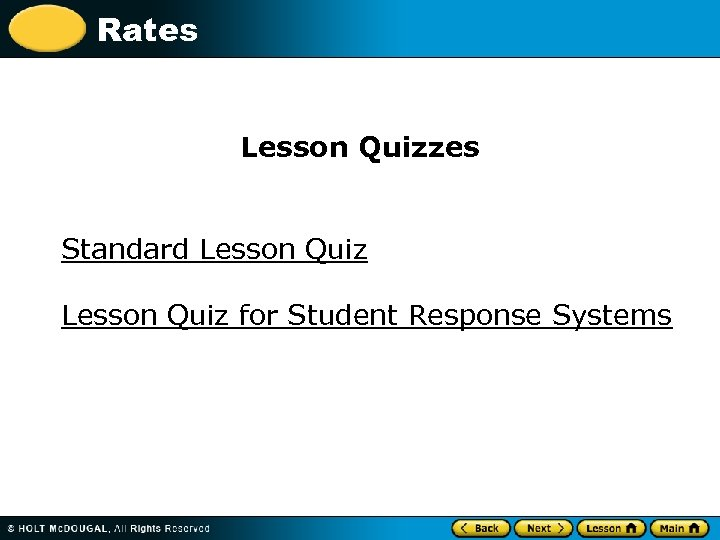Rates Lesson Quizzes Standard Lesson Quiz for Student Response Systems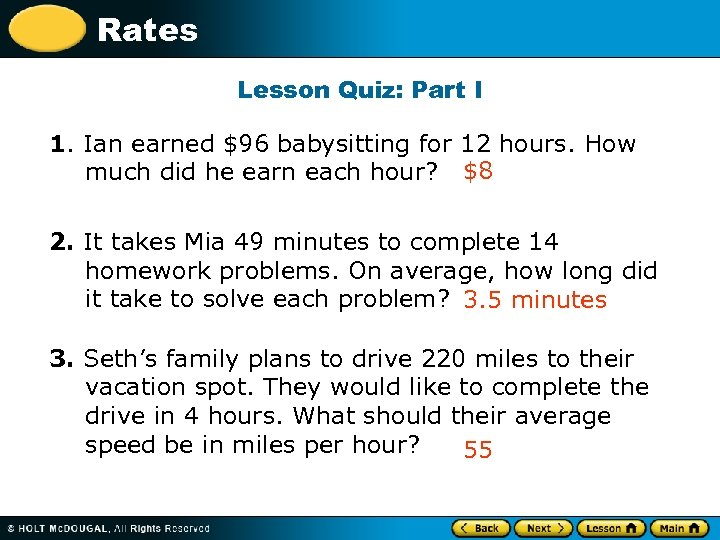Rates Lesson Quiz: Part I 1. Ian earned \$96 babysitting for 12 hours. How much did he earn each hour? \$8 2. It takes Mia 49 minutes to complete 14 homework problems. On average, how long did it take to solve each problem? 3. 5 minutes 3. Seth’s family plans to drive 220 miles to their vacation spot. They would like to complete the drive in 4 hours. What should their average speed be in miles per hour? 55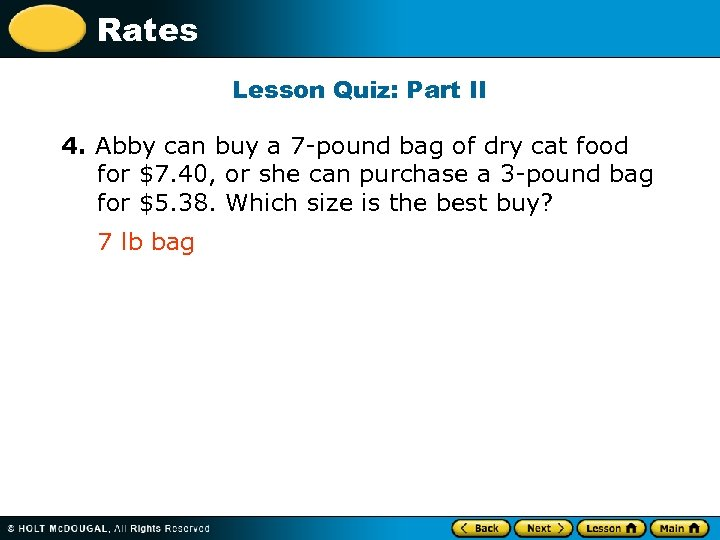Rates Lesson Quiz: Part II 4. Abby can buy a 7 -pound bag of dry cat food for \$7. 40, or she can purchase a 3 -pound bag for \$5. 38. Which size is the best buy? 7 lb bag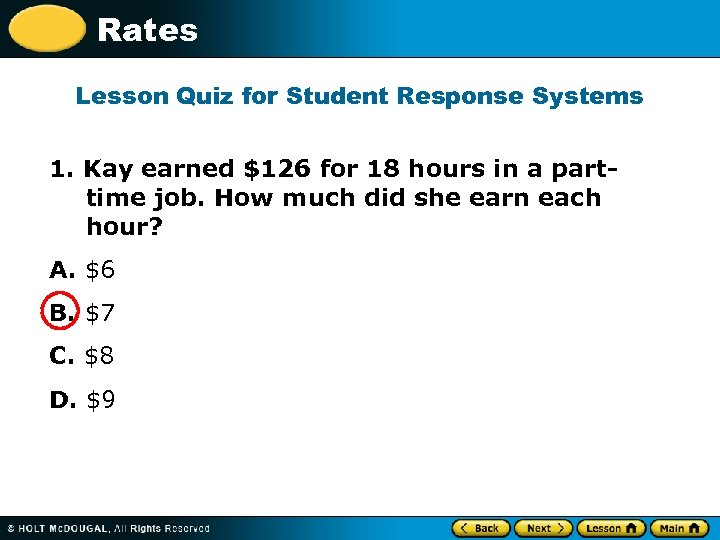Rates Lesson Quiz for Student Response Systems 1. Kay earned \$126 for 18 hours in a parttime job. How much did she earn each hour? A. \$6 B. \$7 C. \$8 D. \$9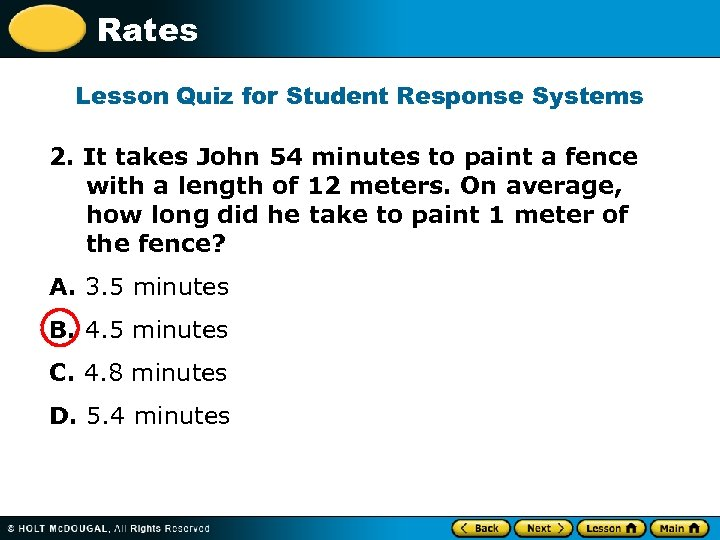Rates Lesson Quiz for Student Response Systems 2. It takes John 54 minutes to paint a fence with a length of 12 meters. On average, how long did he take to paint 1 meter of the fence? A. 3. 5 minutes B. 4. 5 minutes C. 4. 8 minutes D. 5. 4 minutes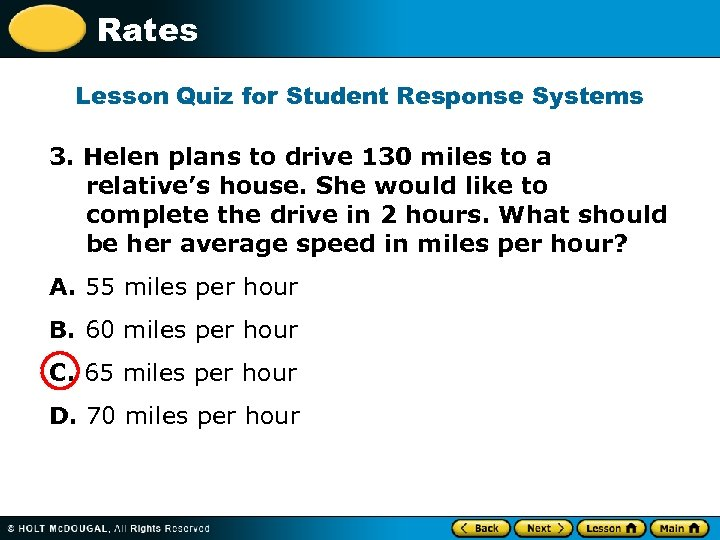Rates Lesson Quiz for Student Response Systems 3. Helen plans to drive 130 miles to a relative’s house. She would like to complete the drive in 2 hours. What should be her average speed in miles per hour? A. 55 miles per hour B. 60 miles per hour C. 65 miles per hour D. 70 miles per hour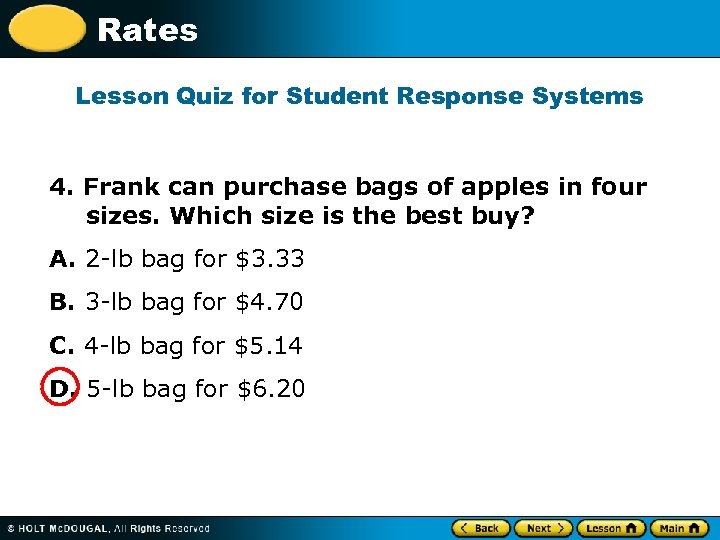Rates Lesson Quiz for Student Response Systems 4. Frank can purchase bags of apples in four sizes. Which size is the best buy? A. 2 -lb bag for \$3. 33 B. 3 -lb bag for \$4. 70 C. 4 -lb bag for \$5. 14 D. 5 -lb bag for \$6. 20